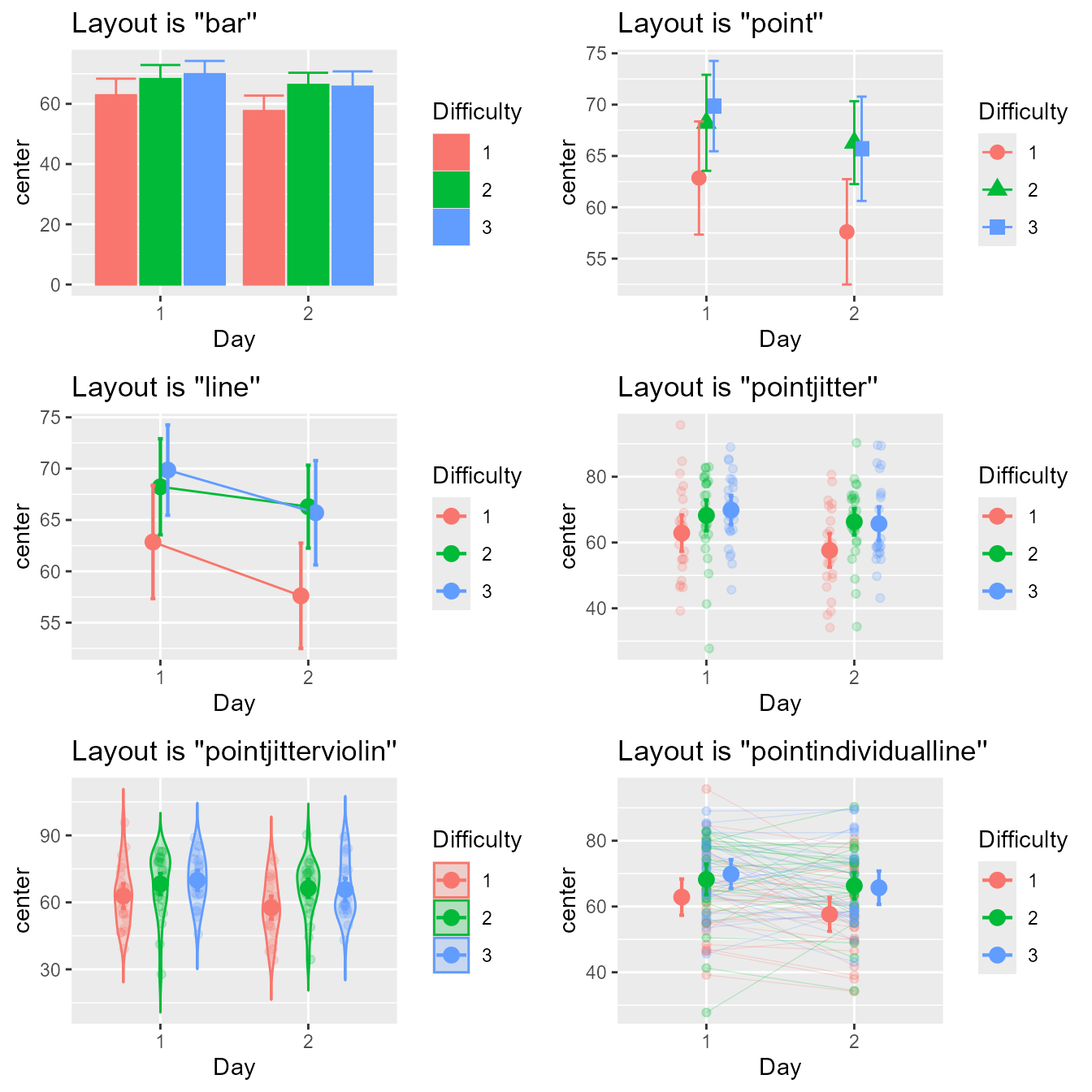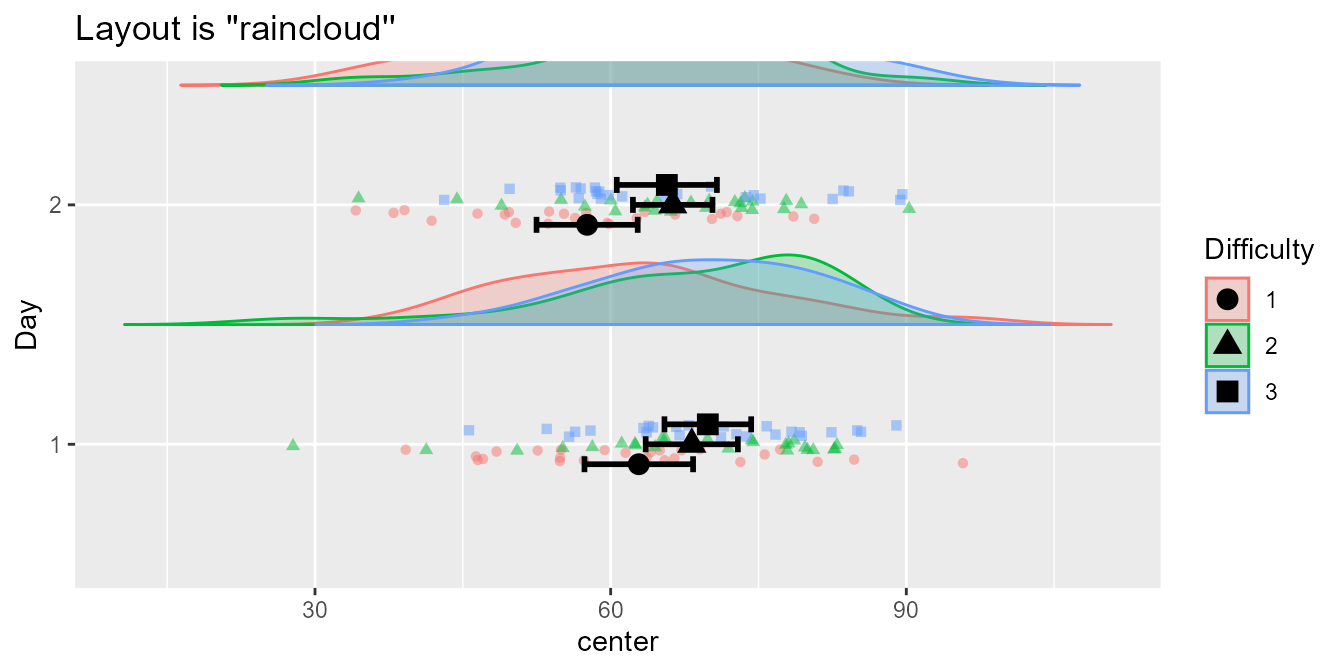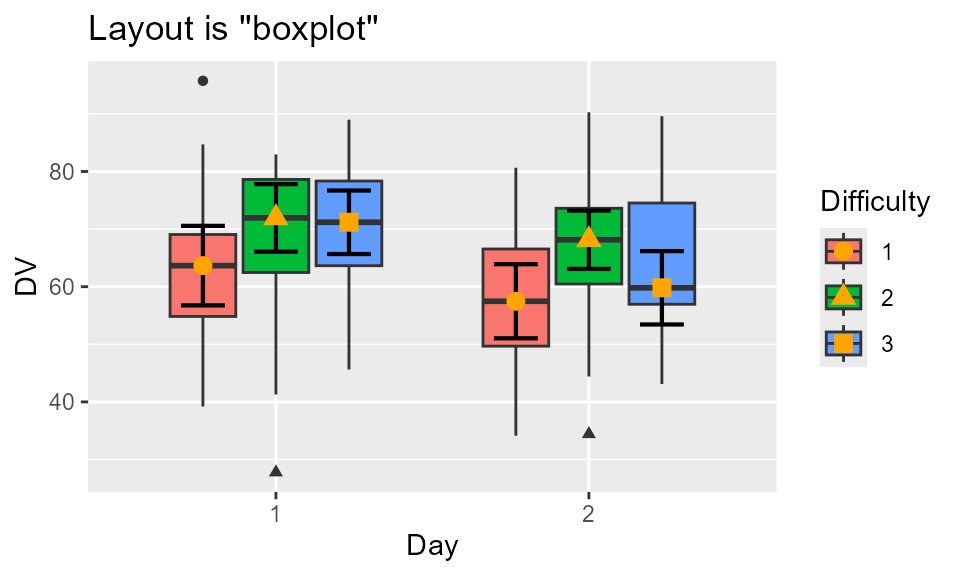superbPlot() comes with seven built-in layouts for plotting your data. However, it is possible to add additional, custom-made layouts. In this vignette, we present rapidly the existing layouts, then show how to supplement superb with your own layouts.

## The built-in plot layouts

When calling superbPlot(), you use the plotStyle = "layout" option to indicate which layout you wish to use. Internally, superbPlot() is calling a function whose name is superbPlot."layout"(). For example, with plotStyle = "line", the plot is actually performed by the function superbPlot.line().

The seven layout available in superbPlot package are :

• superbPlot.line() : shows the results as points and lines,

• superbPlot.lineBand(): Shows the confidence intervals as a semi-transparent band rather than with bars.

• superbPlot.point(): shows the results as points only,

• superbPlot.bar() : shows the results using bars,

• superbPlot.pointjitter(): shows the results with points, and the raw data with jittered points,

• superbPlot.pointjitterviolin(): also shows violin plot behind the jitter points, and

• superbPlot.pointindividualline(): show the results with fat points, and individual results with thin lines,

• superbPlot.raincloud(): Shows the results with distribution and jitter.

To determine if a certain function is superbPlot-compatible, use the following function:

superb:::is.superbPlot.function("superbPlot.line")
##  TRUE

where you put between quote the name of a function. When devising your own, custom-made function, it is a good thing to check that it is superbPlot-compatible.

## Illustrating some of the built-in layouts

To get a sense of the currently available layouts, we first generate a dataset composed of randomly generated scores mimicking a 3 $$\times$$ 2 design with three degrees of Difficulties (as a between-group factor) and two days of testing (as a within-subject factor). It is believed (and simulated) that all two factors have main effets on the scores.

testdata <- GRD(
RenameDV   = "score",
SubjectsPerGroup = 25,
BSFactors  = "Difficulty(3)",
WSFactors  = "Day(day1, day2)",
Population = list(mean = 65,stddev = 12,rho = 0.5),
Effects    = list("Day" = slope(-5), "Difficulty" = slope(3) )
)
head(testdata)
##   id Difficulty score.day1 score.day2
## 1  1          1   76.06188   41.96855
## 2  2          1   30.20333   60.88342
## 3  3          1   53.68103   55.13152
## 4  4          1   42.07037   45.08207
## 5  5          1   50.52381   43.20711
## 6  6          1   87.16833   68.49903

For simplicity, we define a function whose arguments are the dataset and the layout:

mp <- function(data, style, ...) {
superbPlot(data,
WSFactors = "Day(2)",
BSFactors = "Difficulty",
variables = c("score.day1", "score.day2"),
adjustments = list(purpose="difference", decorrelation="CM"),
plotStyle = style,
...
)+labs(title = paste("Layout is ''",style,"''",sep=""))
}

Lets compute the plots will the first six built-in layouts and show them

p1 <- mp(testdata, "bar")
p2 <- mp(testdata, "point")
p3 <- mp(testdata, "line")
p4 <- mp(testdata, "pointjitter" )
p5 <- mp(testdata, "pointjitterviolin")
p6 <- mp(testdata, "pointindividualline")

library(gridExtra)
grid.arrange(p1,p2,p3,p4,p5,p6,ncol=2)Figure 1a. Look of the six built-in layouts on the same random dataset

The last format, a raincloud plot (Allen et al., 2021), is better seen with coordinates flipped over:

mp(testdata, "raincloud") + coord_flip()Figure 1b. The seventh layout, the raincloud

For more controls, you can manually set the colors, the fills and/or the shapes, as done here in a list:

ornate = list(
scale_colour_manual( name = "Difference",
labels = c("Easy", "Hard", "Unthinkable"),
values = c("blue", "black", "purple")) ,
scale_fill_manual( name = "Difference",
labels = c("Easy", "Hard", "Unthinkable"),
values = c("blue", "black", "purple")) ,
scale_shape_manual( name = "Difference",
labels = c("Easy", "Hard", "Unthinkable") ,
values = c(0, 10, 13)) ,
theme_bw(base_size = 9) ,
labs(x = "Days of test", y = "Score in points" ),
scale_x_discrete(labels=c("1" = "Former day", "2" = "Latter day"))
)
library(gridExtra)
grid.arrange(
p1+ornate, p2+ornate, p3+ornate,
p4+ornate, p5+ornate, p6+ornate,
ncol=2)Figure 2a. The six built-in template with ornamental styling added.

These are just a few examples. However, if these layouts do not fit yours needs, it is possible to devise a custom-made layout and inform superbPlot to use it. To that end, see the instructions below.

## Devising a custom-made plot layout

In a nutshell, the purpose of superbPlot() is to

• compile the summary information (location of the summary statistic, upper width and lower width of the interval) and that, for each level of the factors;

• applies all the adjustments needed in producing the summary;

• and finally, calls a plot function accepting pre-defined arguments

In devising your own plot function, it is important that (i) the function name begins with superbPlot.; (ii) the function accept very specific arguments with very precise names.

Here is the header for a function corresponding to a plot style called, say, foo (plotStyle = "foo"):

superbPlot.foo <- function(
summarydata,
xfactor,
groupingfactor,
rawdata
# any optional argument you wish
) {
plot <- ggplot() ## ggplot instructions...
return(plot)
}

In what follow, it is assumed that one factor is placed on the horizontal axis (xfactor), another one is used to group the point (groupingfactor), and up to two additional factors will results in columns and rows of panels (addfactors; of course, in devising your own template, you may use different placement). superbPlot() is restricted to a maximum of four factors.

The arguments are:

• summarydata: this data frame will contain the column center indicating the statistic’s value, lowerwidth and upperwidth indicating how many units below and above center the error bar extends. The data frame will also have columns for all the factors, and there will be as many lines as there are combinations of factors.

• xfactor is the factor to put on the horizontal axis;

• groupingfactor is the factor used to create groups of points;

• addfactors are up to two additional factors to create the rows and columns of panels. addfactors is formatted for facetting (e.g., for factors “A” and “B,” addfactors would be “A~B”);

• rawdata : this data.frame contains the raw data with factors being transformed as.factor and the dependent column being renamed DV. When the data are in wide format, rawdata is reshaped to long format.

• {optional arguments} can be used. They must be named here; when calling superbPlot(), any argument whose name match your optional argument will be transmitted to your custom-made function.

## The simplest example

What follow is a simple example that will design a template that we will call simple. This layout will display the descriptive statistics and error bars. Everything will be black and white (no color instruction) and superimposed (no grouping instruction).

The result will be:Figure 3. Mean score with 95% confidence interval using the simple plot layout.

To make this plot, we design a function superbPlot.simple as:

superbPlot.simple <- function(
summarydata, xfactor, groupingfactor, addfactors, rawdata
) {
plot <- ggplot(
data = summarydata,
mapping = aes_string( x = xfactor, y = "center", group=groupingfactor)
) +
geom_point(  ) +
geom_errorbar( mapping = aes_string(ymin = "center + lowerwidth",
ymax = "center + upperwidth")  )+

return(plot)
}

The first instruction, ggplot defines the source data to be summarydata with horizontal axis being in the string xfactor (this is the reason that mapping must be given with aes_string). The position of the descriptive statistics is automatically computed and stored in a column called "center".

The second instruction put points for each "center", and the third instruction places error bars. In that case, the ymin and ymax information are contained in center+lowerwidth and center+upperwidth where lowerwidth and upperwidth are automaticall computed and stored in the summarydata dataframe.

The last instructions generates distinct panels for each level of the remaining factors.

You can check that this function is superbPlot-compatible with:

superb:::is.superbPlot.function("superbPlot.simple")
##  TRUE

If TRUE, then we are ready to use this layout, here with the demo dataset TMB1964r whose result was shown above in Figure 3:

superbPlot(TMB1964r,
WSFactors = "T(7)",
BSFactors = "Condition",
variables = c("T1","T2","T3","T4","T5","T6","T7"),
plotStyle = "simple"
)

## Optional arguments

The above simple layout does not accept optional arguments. To integrate optional arguments, one method is to insert graphic directives inside the layers, e.g., inside geom_point.

A convenient method is with do.call and modifyList, for example:

    do.call( geom_point, modifyList(
list( size= 3 ##etc., the default directives##
), myownParams
))

A full example it therefore

superbPlot.simpleWithOptions <- function(
summarydata, xfactor, groupingfactor, addfactors, rawdata,
myownParams = list()  ## will be used to add the optional arguments to the function
) {
plot <- ggplot(
data = summarydata,
mapping = aes_string( x = xfactor, y = "center", group="Condition")
) +
do.call( geom_point, modifyList(
list( color ="black" ),
myownParams
)) +
do.call( geom_errorbar, modifyList(
list( mapping = aes_string(ymin = "center + lowerwidth",
ymax = "center + upperwidth")  ),
myownParams
)) +

return(plot)
}
superb:::is.superbPlot.function("superbPlot.simpleWithOptions")
##  TRUE
superbPlot(TMB1964r,
WSFactors = "T(7)",
BSFactors = "Condition",
variables = c("T1","T2","T3","T4","T5","T6","T7"),
plotStyle = "simpleWithOptions",
## here goes the optional arguments
myownParams = list(size=1, color="purple", position = position_dodge(width = 0.3) )
)

In that example, the same parameters are sent to geom_point and to geom_errorbar. It is left as an exercice to the reader to use two distinct sets of optional parameters, one for the points, the other for the error bars.

## Getting feedback information

It is sometimes useful to extract variables out of the function when debugging the code. A useful function is to use runDebug(). This function (shipped with suberb) will display text and transfer any variables you want into the global environment.

options(superb.feedback = 'all')
runDebug( 'where are we?', "Text to show when we get there",
c("variable1", "variable2", "etc"),
list( "var1InTheFct", "var2InTheFct", "varetcInTheFct")
)
## ==> Text to show when we get there <==
##     variables dumped in: variable1, variable2, etc

For example, the following will get the dataframes:

superbPlot.empty <- function(
summarydata, xfactor, groupingfactor, addfactors, rawdata
) {
runDebug( 'inempty', "Dumping the two dataframes",
c("summary","raw"), list(summarydata, rawdata))

plot <- ggplot() # an empty plot
return(plot)
}
options(superb.feedback = 'inempty')  ## turn on feedback when reaching 'inempty'
superbPlot(TMB1964r,
WSFactors = "T(7)",
BSFactors = "Condition",
variables = c("T1","T2","T3","T4","T5","T6","T7"),
plotStyle = "empty"
)
## ==> Dumping the two dataframes <==
##     variables dumped in: summary, raw

You see Dumping the two dataframes followed by summary and raw. These two variables are now in the global environment and you can manipulate them. You can also use them in testing your plotting functions, for example

superbPlot.simple(summary, "T", "Condition", ".~.", raw)

## An example

In what follow, we create a toy example where the raw data will be shown with smileys. Note that this example may not work in Rstudio (see “limitation” on emojifont page )

We first need the emojifont library

# install.packages("emojifont")
library(emojifont)

Then we define a "smiley" layout where the emojis are shown with geom_text layer:

superbPlot.smiley <- function(
summarydata, xfactor, groupingfactor, addfactors, rawdata
) {
# the early part bears on summary data with variable "center"
plot <- ggplot(
data = summarydata,
mapping = aes_string(
x = xfactor, y = "center",
fill = groupingfactor,
shape = groupingfactor,
colour = groupingfactor)
) +
geom_point(position = position_dodge(width = .95)) +
geom_errorbar( width = .6, position = position_dodge(.95),
mapping = aes_string(ymin = "center + lowerwidth", ymax = "center + upperwidth")
)+
# this part bears on the rawdata only with variable "DV"
geom_text(data = rawdata,
position = position_jitter(0.5),
family="EmojiOne", label=emoji("smile"), size=6,
mapping=aes_string(x=xfactor, y="DV", group = groupingfactor)
) +

return(plot)
}

We check that it is a superbPlot-compatible function:

superb:::is.superbPlot.function("superbPlot.smiley")
##  TRUE

It is all we need! It can be inserted in a superbPlot call:

superbPlot(TMB1964r,
WSFactors = "T(7)",
BSFactors = "Condition",
variables = c("T1","T2","T3","T4","T5","T6","T7"),
plotStyle = "smiley"
)Figure 5. smile!